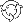The Theory \ Details \ general information \ types of returns

# General Information

## Types of returns

Consider some asset(is allowed to denote investor’s portfolio wealth as well)over the periodto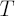. Timeis measured in years. Let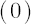and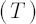denote prices ofat timeandrespectively.
Below are presented the definitions actively used in the current document. The corresponding terminology is not settled yet; therefore the divergences with the terms used in other sources might occur.

### Types of Returns

Various definitions of return serve to measure the degree of price change over the given time period.
Definition. Quantityis called Simple return or Arithmetic return over the periodto.
Definition. Quantity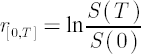is called Log return or Geometric return over the periodto.

Note. Applying Taylor series expansion up to fourth-order term, one can obtain the following approximation of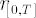when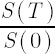is close to### Rates of Return

Under Rates of return we will further denote returns that are normalized to annual basis. Rates of return may also be called Annualized returns or simply Annual returns. Another frequently used synonym is Growth Rate.
Definition. Quantitywill be called Simple rate of return (other notations include Arithmetic rate of return or Rate of return without compounding) over the periodto.
Definition. Quantity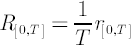will be called Logarithmic rate of return (
Geometric rate of return or Continuously compounded rate of return respectively) over the periodto.

Important! For simplicity reasons everywhere hereinafter under the terms rate of return and growth rate a logarithmic rate of return will be implied. Then, when one requires using simple rates of return, the latter will be indicated explicitly.

### Expected Rates of Return

The symbol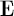below stands for Mathematical Expectation (averaging over all possible outcomes with weights equal to respective probabilities).

Definition. Quantity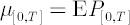will be called Expected simple rate of return (
Expected arithmetic rate of return or Expected rate of return without compounding) over the periodto.

Note. Maximization of portfolio expected rate of return is equivalent to the maximization of expected portfolio wealth.